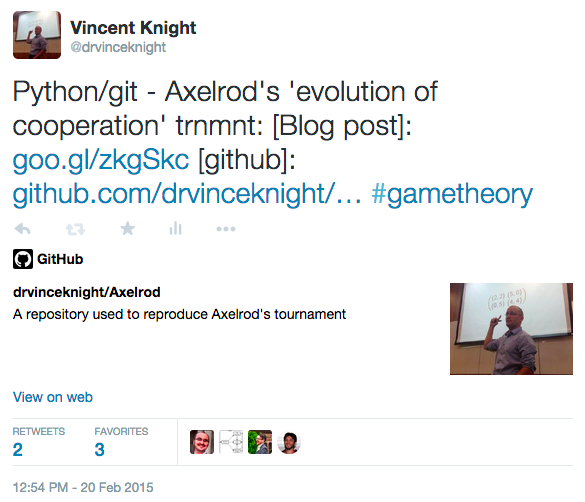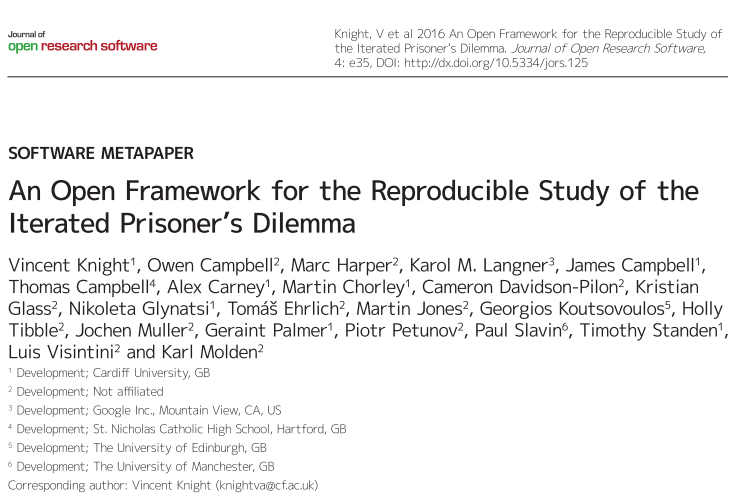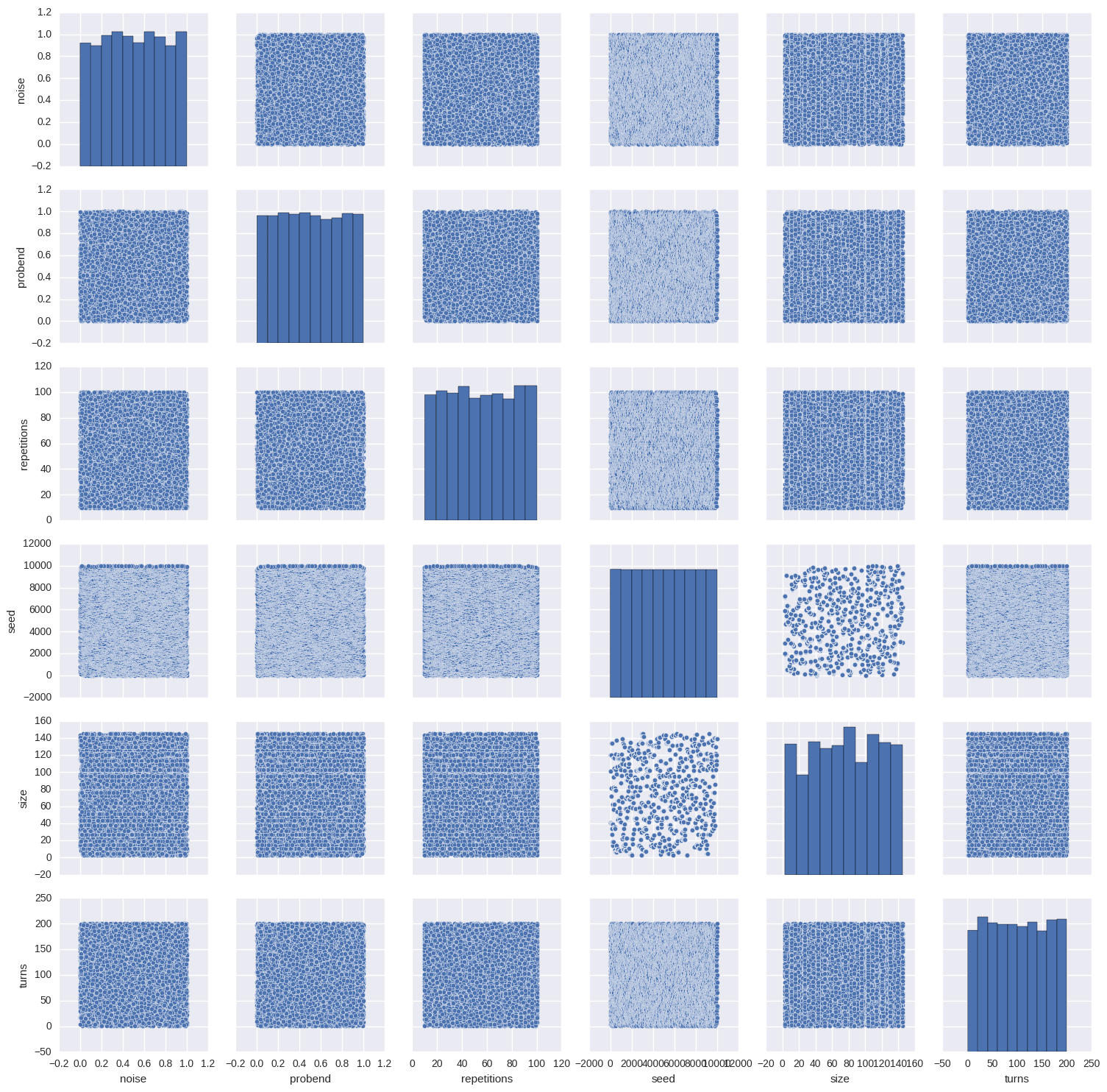### The Iterated Prisoner's Dilemma and the Axelrod Library

## Themes

reveal.js comes with a few themes built in:
Black (default) - White - League - Sky - Beige - Simple
Serif - Night - Moon - Solarized

$$\begin{pmatrix} 3,3&0,5\\ 5,0&1,1 \end{pmatrix}$$

'This course has taught me to not trust my classmates.'
1. Robert Axelrod
2. 1980a: 14+1 strategies
3. 1980b: 64+1 strategiesclass TitForTat(Player):
"""A player starts by cooperating and then mimics previous move by opponent."""

name = 'Tit For Tat'
classifier = {
'memory_depth': 1,  # Four-Vector = (1.,0.,1.,0.)
'stochastic': False,
'inspects_source': False,
'manipulates_source': False,
'manipulates_state': False
}

@staticmethod
def strategy(opponent):
return 'D' if opponent.history[-1:] == ['D'] else 'C'


class TestTitForTat(TestPlayer):

name = "Tit For Tat"
player = axelrod.TitForTat
expected_classifier = {
'memory_depth': 1,
'stochastic': False,
'inspects_source': False,
'manipulates_source': False,
'manipulates_state': False
}

def test_strategy(self):
"""Starts by cooperating."""
self.first_play_test(C)

def test_effect_of_strategy(self):
"""Repeats last action of opponent history."""
self.markov_test([C, D, C, D])
self.responses_test([C] * 4, [C, C, C, C], [C])
self.responses_test([C] * 5, [C, C, C, C, D], [D])## Demo### Outcomes• Convex programming applied to the PD
• Meta study of tournaments
• Machine learning strategies

Press and Dyson (2012): "Iterated Prisoner's Dilemma contains strategies that dominate any evolutionary opponent."

$$p = (P(C|CC), P(C|CD), P(C|DC), P(C|DD))$$

Optimise: $\frac{x^TQx+xc^t}{x^TQ'x+xc'^T}$Lee, Harper and Dyer (2015): "The Art of War: Beyond Memory-one Strategies in Population Games"

Axelrod-dojo